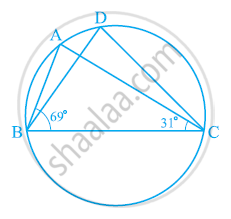# In the given figure, ∠ABC = 69°, ∠ACB = 31°, find ∠BDC. - Mathematics

In the given figure, ∠ABC = 69°, ∠ACB = 31°, find ∠BDC.#### Solution

In ΔABC,

∠BAC + ∠ABC + ∠ACB = 180° (Angle sum property of a triangle)

⇒ ∠BAC + 69° + 31° = 180°

⇒ ∠BAC = 180° − 100º

⇒ ∠BAC = 80°

∠BDC = ∠BAC = 80° (Angles in the same segment of a circle are equal)

Is there an error in this question or solution?
Chapter 10: Circles - Exercise 10.5 [Page 185]

#### APPEARS IN

NCERT Class 9 Maths
Chapter 10 Circles
Exercise 10.5 | Q 4 | Page 185

Share